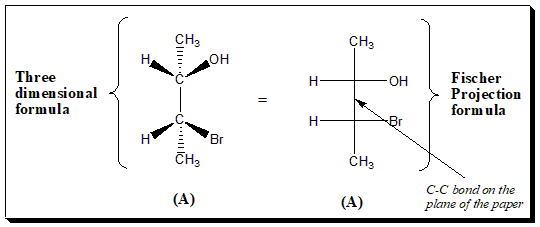# Fischer Projection: hassle free way to depict a stereoformula in 2D projection

One of the major problems in organic chemistry is the representation of three-dimensional structures in a two-dimensional media (viz. sheet of paper, blackboard, etc.). Chemists sometimes represent structures for chiral molecules with two-dimensional formulas called Fischer projection formulas. These two-dimensional formulas are a quick way to show three dimensions without the hassle of having to draw 3-D.

The Fischer projection, devised by the carbohydrate chemist Emil Fischer in 1891. This projection formula is a technique to represent three-dimensional organic molecules in two-dimensional structures on a two-dimensional medium such as a paper. a black board, etc. Fischer projections were originally proposed for the depiction of carbohydrates, amino acids and used by chemists, particularly in organic chemistry and biochemistry.

Horizons and vertical lines are employed to represent the bonds and the crossing of a horizontal and a vertical line represents the carbon atom. If Fischer projections are used certain rules must be obeyed and certain conventions clearly understood, otherwise the structure will be misinterpreted and can easily lead to incorrect conclusions.

Rule 1:

Let us take glyceraldehyde with one chiral center as an illustrative example. The structure is written with the longest carbon chain vertically rather than horizontal form and the carbon atoms bearing the lower number (in standard nomenclature terms) is normally written uppermost. In Glyceraldehyde, -CHO is placed at the top of the Fischer projection because this is C(1) according to Nomenclature rules.

Note: Vast majority of the cases the carbon in the highest oxidation state is positioned at the top of the diagram.

Rule 2:

In a Fisher projection the chiral atom under consideration lies in the plane of the paper and although all bonds represented by plain lines it is understood that groups vertically linked to the chiral atom project below the plane of the paper and the groups horizontally linked to the chiral atom project above the paper. [In other words, Vertical lines represent bonds that project behind the plane of the paper (or that lie in it). Horizontal lines represent bonds that project out of the plane of the paper.] This must be clearly understood when visualizing Fisher projections. When you look at the diagram the horizontal lines represent atoms that are coming out at you.  The vertical lines mean they are going away from you.

Two chiral center

Another example of a chiral molecules having two chiral centers depicting three dimensional formula and Fischer projection formula. This to relate how stereo-structure and 2D projection relate to each other.

Rule 3: Fischer Projection Manipulation

For purpose of comparison a Fisher projection may be rotated through 180º in the plane of the paper but no other manipulation is permitted. Fischer projections can be rotated 180 degrees and still be the same. However, if you flip horizontally or vertically, it becomes the enantiomer.

The following operations result in an alternative projection of the same molecule:

The following operations lead to mirror image projections:

1. Interchange of any two ligands / Flipping

Illustration to explain the manipulation of Fischer projection formulae

Horizontal Flip

To understand this let us take a simple molecule with two chiral centers. The Fischer projection (1) has been flipped horizontally resulting in (2), where the configuration at both the chiral centers is reversed.  Thus (1) and (2) are enantiomers of each other.  The first projection has an S, R configuration.  The second projection has an R, S configuration.

Vertical Flip

Now let’s look at a vertically flipped diagram. These compounds are enantiomers of each other. Again configuration at both the chiral centers is reversed.

180 degree rotation

Finally, notice what happens when the diagram/Fischer projection is rotated 180º in the plane of the paper. Fischer projections can be rotated 180 degrees and still be the same.

Note: Beginners who have difficulty in mentally imagining flipping and rotations of molecules may use molecular models (may be “ball and stick”) and learn.

References

Solomons’ Organic Chemistry, T. W. Graham SolomonsCraig B. FryhleScott A. Snyder, Wiley, New York, 2017.

Jonathan Clayden,  Nick GreevesStuart Warren, Organic Chemistry. Oxford University Press, New York, 2014.

Bernard Testa, Principles of organic stereochemistry, Marcel Dekker Inc., New York, 1979.

### 2 thoughts on “Fischer Projection: hassle free way to depict a stereoformula in 2D projection ”

1.Chandramouli R

Thanks for this post.
Request a video demonstration of Fischer projections would help beginners like us 🙂